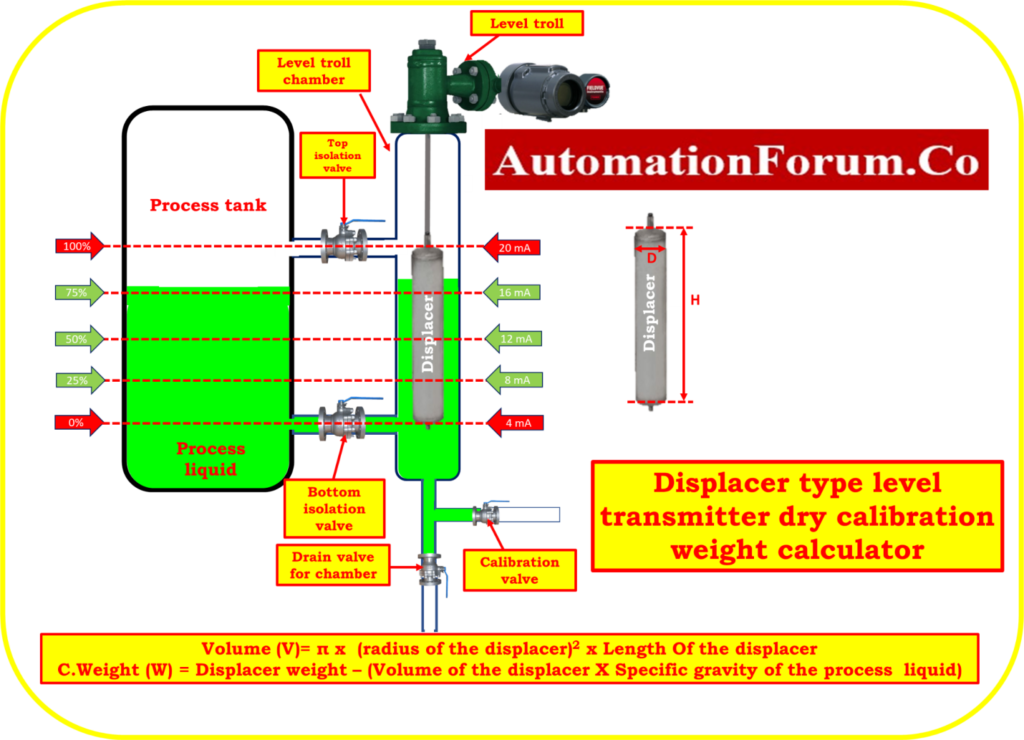# Displacer type level transmitter dry calibration weight calculator## Significance of dry calibration weight calculator

The Displacer level transmitter dry calibration weight calculator is a valuable tool used in industrial settings for the calibration of displacer level transmitters without the need for liquid. It provides several important benefits:

• The calculator ensures precise calibration by determining the appropriate weight values for simulating liquid levels.
• This accuracy helps maintain the reliability and performance of displacer level transmitters, ensuring accurate measurements and proper operation.
• Dry calibration eliminates the need for liquid handling, reducing time and costs associated with preparing and disposing of liquids during calibration.
• The calculator simplifies the calibration process by directly providing the weight values required, saving time and resources.
• Dry calibration allows for calibration in situations where liquid calibration may be impractical or not feasible.
• It offers the flexibility to calibrate displacer level transmitters in various environments, including hazardous or inaccessible locations, where liquid calibration may be challenging or restricted.

## How do you calculate displacer weight?

To find the calibration weight values for a displacer-type level control, you need to consider the specific gravity of the liquid, the displacer dimensions, and the calibration requirements.

Here’s a general procedure to determine the calibration weight values:

## Identify the specific gravity of the liquid:

The specific gravity represents the density of the liquid compared to the density of water. It is typically provided by the liquid manufacturer or can be measured using a hydrometer or similar instrument.

## Determine the displacer characteristics:

Note the dimensions of the displacer, such as the diameter and length. These dimensions are important for calculating the displacer’s volume and weight.

## Calculate the volume of the displacer:

The volume of the displacer can be calculated using the appropriate formula based on its shape. For example, if the displacer is cylindrical, you can use the formula for the volume of a cylinder:

Make sure the units are consistent (e.g., inches or centimeters).

## Calculate the weight values:

Multiply the volume of the displacer by the specific gravity of the liquid and Subtract from the displacer weight to obtain the weight value. This will provide the weight required to simulate the desired liquid level.

## Formula for calculating the volume of displacer

Volume = π x  (radius of the displacer)2 x Length Of the displacer.

## Formula for calculating the displacer weight loss due to process liquid

Displacer weight loss due to process liquid =Volume of the displacer X Specific gravity of the process  liquid

## Formula for calculating the calibration weight

Weight = Displacer weight – (Volume of the displacer X Specific gravity of the process  liquid)

## How do you calculate displacer weight?

Displacer and process liquid characteristics:

W = Displacer weight in 2400 Gram

D = Displacer diameter is 7.0 Centimeter

H = Length of Displacer is 32.42 Centimeter

Sg = Specific gravity of process liquid is 1.1

V = Volume of displacer.

So,

Radius of the displacer r = 7.0/2 = 3.5 centimeters

Displacer Actual volume V = πr2h

Volume V = 3.14 × r² × h

= 3.14 × 3.5 × 3.5 × 32.42 =  1247.04 cubic centimeter

## Zero calibration weight value calculation (0%)

Attach a displacer or 2400 gramme weight that is equal to the displacer to the torque tube arm rod of the transmitter for zero percentage calibration weight calculating.

## Span calibration weight value calculation (100%)

The weight value can be calculated using the formula:

Displacer weight loss due to process liquid =Volume of the displacer X Specific gravity of the process  liquid)

Weight = Displacer weight – (Volume of the displacer X Specific gravity of the process  liquid)

Weight = 2400 – (1247.04 x 1.1)

=2400 – 1371.744

Span calibration weight = 1028.26 grams

## Weight for each 25% calibration check of linearity

For each 25% = Displacer weight loss due to process liquid/4

= (1371.744)/4

For each 25% = 342.936 grams